Courses

# Revisal Problems (Past 13 Years) JEE Main (Structure Of Atom)

## 24 Questions MCQ Test Chemistry for JEE Advanced | Revisal Problems (Past 13 Years) JEE Main (Structure Of Atom)

Description
This mock test of Revisal Problems (Past 13 Years) JEE Main (Structure Of Atom) for JEE helps you for every JEE entrance exam. This contains 24 Multiple Choice Questions for JEE Revisal Problems (Past 13 Years) JEE Main (Structure Of Atom) (mcq) to study with solutions a complete question bank. The solved questions answers in this Revisal Problems (Past 13 Years) JEE Main (Structure Of Atom) quiz give you a good mix of easy questions and tough questions. JEE students definitely take this Revisal Problems (Past 13 Years) JEE Main (Structure Of Atom) exercise for a better result in the exam. You can find other Revisal Problems (Past 13 Years) JEE Main (Structure Of Atom) extra questions, long questions & short questions for JEE on EduRev as well by searching above.
QUESTION: 1

### Direction (Q. Nos. 1-24) This section contains 24 multiple choice questions. Each question has four choices (a), (b), (c) and (d), out of which ONLY ONE option is correct. Q. Wavelength associated with water molecule moving with a velocity of 500 ms-1 is

Solution: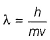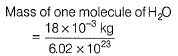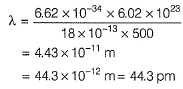QUESTION: 2

### Assume H-atom (rH = 0.0529nm) and proton (rp =1.5x 10-15m)to be spherical. Fraction of the space in an atom of hydrogen that is occupied by the nucleus is

Solution:

Proton is a part of nucleus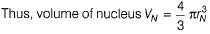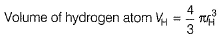Fraction of the volume of hydrogen occupied by nucleus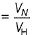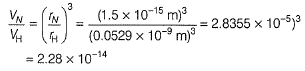QUESTION: 3

### What electronic transition in a hydrogen atom, starting from the orbit n = 7 will produce infrared light of wavelength 2170 nm?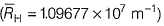Solution: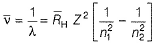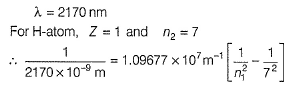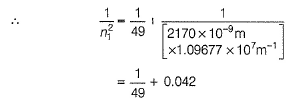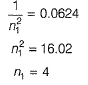QUESTION: 4

If for a given value of n, perimissible values of azimuthal quantum number l be 0,1,2...... n, then magnetic moment of chromium (24) would be

Solution:

[Cr] = [Ar]3d54s1

Using this we get,

μ = √(n(n + 2)) = √(6(6 + 2)) = √48 BM

Hence D

QUESTION: 5

Velocity of the electron in H-atom in second orbit is

Solution:

Angular momentum is quantised, thus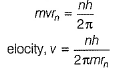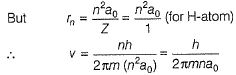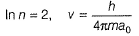QUESTION: 6

If uncertainties in the measurements of position and momentum are equal, then uncertainty in the measurement of velocity is

Solution:

By H eisenberg’s uncertainty principle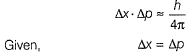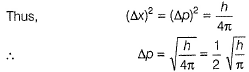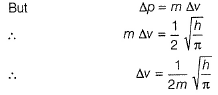QUESTION: 7

For the two moving particles A and B, following values are given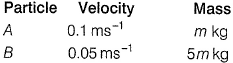Q. Ratio of de-Broglie wavelength associated with the particles A and B is

Solution:

de-Broglie wavelength is given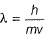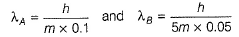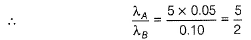QUESTION: 8

The wave number of the spectral line in the emission spectrum of H-atom will be equal to 8/9 times the Rydberg’s constant if the electron jumps from

Solution:

Wave number of the spectral line in H-atom is given by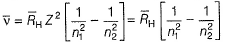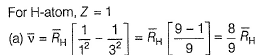QUESTION: 9

Which one of the following constitutes a group of the isoelectronic species?

Solution: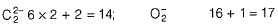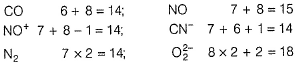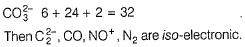QUESTION: 10

In an experiment reproducing the measurements of Rutherford and his co-workers, 22 x10-3 mg of He gas was collected in one year from a sample of radium. This sample was observed to emit 1. 06 x 1011 α - particlesper second. Thus, Avogadro’s number is

Solution:

22 x 10-3 mg per year = 22 x 10-6 g per year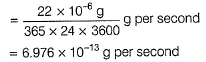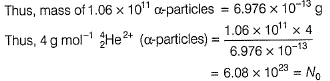QUESTION: 11

Medical experts generally consider a lead level of 30 μg Pb per (dL) of blood to pose a significant health risk (Pb =208). Number of lead atoms per cm3 of blood is

Solution: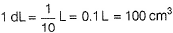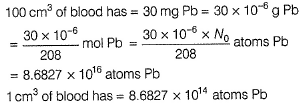QUESTION: 12

In Schrodinger wave equation given below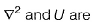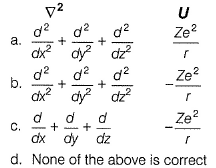Solution: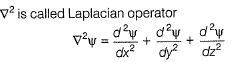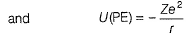QUESTION: 13

Which hydrogen like species will have same radius as that of Bohr’s orbit of hydrogen atom?

Solution: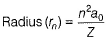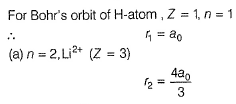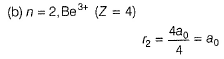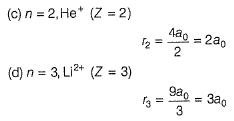QUESTION: 14

An atom for which the electron probability distribution is spherically symmetric about the nucleus is

Solution: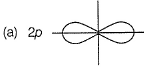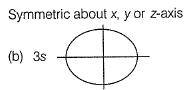(c) 3pas (a)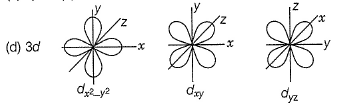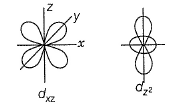QUESTION: 15

Which of the following statements in relation to the hydrogen atom is correct?

Solution: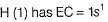Thus, w.r.t. (1s) orbital, 3s, 3p and 3d orbitals will have same energy.

QUESTION: 16

Which of the following options does not represent ground state EC of an atom?

Solution:

Due to extra stability of com ple te ly filled sub orb it or half-filled suborbit
4s13d10 is more stable than 4s23d9. Thus, [Ar] 4s239 is not the ground state electronic configuration.

QUESTION: 17

Statement I :The electronic configuration of N -atom is represented as :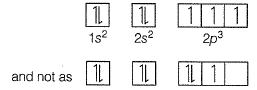Statement II : The electronic configuration of the ground state of an atom is the one which has the greatest multiplicity.

Solution:

N (7) has electronic configuration as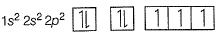Thus, Statement I is correct.
By Hund’s rule of maximum multiplicity, pairing of electrons takes place only after each orbital is half-filled, i.e. maximum multiplicity, and thus, has stable configuration.
Thus, Statement II is also correct and is the correct explanation of Statement I.

QUESTION: 18

If n and l are respectively the principal and azimuthal quantum numbers, then the expression for calculating the total number of electrons in any energy level is

Solution:

For a given value of n
l = 0,1,2......(n - 1)
Total orbitals = (2l + 1)
Total electrons = 2(2l + 1)
Thus, total electrons are obtained by taking sum of all values of l from 0 to (n - 1)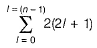QUESTION: 19

3d-suborbit first occurs in fourth orbit. It has

Solution:

For a given orbit, (3d, n = 3, l = 2)
Number of angular nodes = l = 2 for 3d number of radial nodes
= (n — l — 1) = (3 — 2 — 1) = 0
Total nodes = (n - I - 1 + l) = ( n - 1)= 2

QUESTION: 20

How fast is an electron moving if it has a wavelength equal to the distance it travels in one second?

Solution:

Velocity = v cm -1 (say)
Distance travelled in 1s = v cm s-1 x 1 s = v cm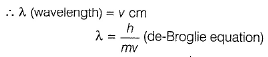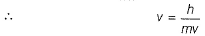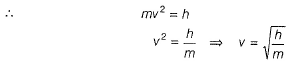QUESTION: 21

In the presence of magnetic field, d-suborbit is

Solution:

In the presence of magnetic field there is splitting of lines (Zeeman’s effect)
Total lines due to splitting = (2l + 1)
Total orbitals =(2l + 1)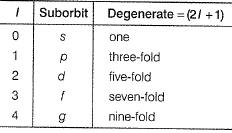QUESTION: 22

Electrons are used to determine the structure of crystal surfaces. To have diffraction, the wavelength λ, of the electrons should be of the order of the lattice constant, which is typically 0.30 nm. What energy do such electrons have?

Solution: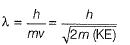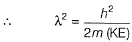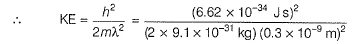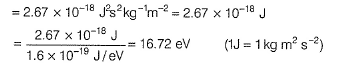QUESTION: 23

The number of d-electrons in Fe2+ (24 electrons) is not equal to

Solution:

Fe = [Ar] 4s23d6
Fe2+ = [Ar]4s03d6
[Electrons of the outermost shell are taken out in the formation of Fe2+ (i.e. 4s)]
Electrons in 3d in Fe2+ = 6
Ne (10) = 1s2,2s2,2p6
p-electrons = 6: Correct
Mg (12)= 1s22s22p63s2
s-electrons = 6: Correct
Fe (26) = 1s22s22p63s23p64s23d6
d-electrons = 6: Correct
Cl (17) = 1s22s22p63s23p5
p-electrons 11 : Incorrect

QUESTION: 24

Bohr's theory is applicable to the following set of species

Solution:

Bohr's theory is applicable to H-atom and FI-like species with one electron.
H (Z =1)1 electron
He+ (Z =2)1 electron
Li2+ (Z = 3) 1 electron
Be3+ (Z = 4)1 electron